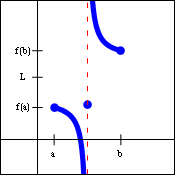## The Intermediate Value Theorem

We already know from the definition of continuity at a point that the graph of a function will not have a hole at any point where it is continuous. The Intermediate Value Theorem basically says that the graph of a continuous function on a closed interval will have no holes on that interval.

### Statement of the Theorem

• Suppose $f(x)$ is continuous on the closed interval   $[a,b]$.   If $L$ is a real number between the values $f(a)$ and $f(b)$, but not equal to either of them, then there exists a number $c$ in the interval   $[a,b]$.   for which   $f(c)=L$.

The graph on the left below illustrates the situation described by the theorem. Since the function $f(x)$ is continuous, the endpoints of the interval are connected by the graph, and the function will pass through all values between $f(a)$ and $f(b)$. The graph on the right below shows a function that is defined everywhere on the closed interval   $[a,b]$,   but is not continuous on that interval. The lack of continuity allows for values between $f(a)$ and $f(b)$ that the function never takes on.### Using the Intermediate Value Theorem

Show that the function   $f(x)=x^{17}-3x^4+14$   is equal to 13 somewhere on the closed interval   $[0,1]$.

 We note that   $f(0)=14$   and   $f(1)=12$, By substituting the endpoints of the closed interval into the function, we obtain the values $f(a)$ and $f(b)$. and   $12 < 13 < 14$. And we note that the value of $L$, 13, does fall between $f(a)$ and $f(b)$. But $f(x)$ is a polynomial and is therefore continuous everywhere, so in particular it is continuous on the closed interval   $[0,1]$. The continuity of the function is the crux of the issue. Without it, the result could not be guaranteed. So we must demonstrate the continuity of the function on the given interval. And since the function is a polynomial, continuity is automatic. Therefore, by the Intermediate Value Theorem, there exists a number $c$ in the interval   $[0,1]$   for which   $f(c)=13$. Having verified all of the hypotheses of the Intermediate Value Theorem, the conclusion must then follow.

### Proof of the Theorem

Even though the statement of the Intermediate Value Theorem seems quite obvious, its proof is actually quite involved, and we have broken it down into several pieces. First, we will discuss the Completeness Axiom, upon which the theorem is based. Then we shall prove Bolzano's Theorem, which is a similar result for a somewhat simpler situation. Then the Intermediate Value Theorem will follow almost immediately.

### The Completeness Axiom

Axioms are statements in a mathematical system that are assumed to be true without proof. The Completeness Axiom is an axiom about the real numbers, and is sometimes phrased in the language of least upper bounds. A real number $x$ is called a least upper bound for a set $S$ if the following two properties are true:

• The number $x$ is an upper bound for the set $S$. (In other words, if $s$ is any number in set $S$, then   $s\le x$.)